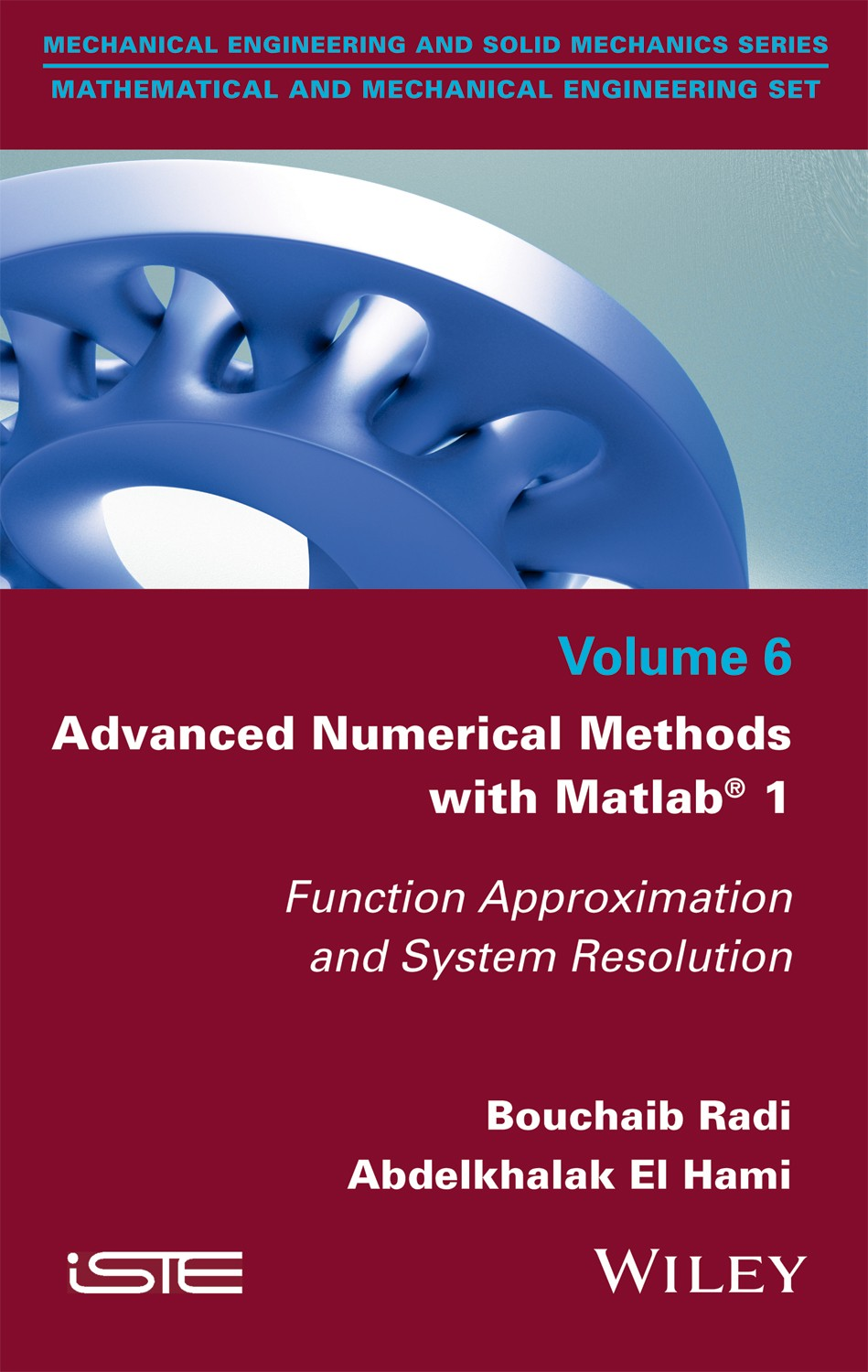pages
238
ISBN
9781786302359

Most physical problems can be written and approximated in the form of mathematical equations (differential, integral, etc.). Mathematicians have always sought to find analytical solutions to the equations encountered in the different engineering sciences (mechanics, physics, biology, automation, etc.). These equations are sometimes so complicated that it takes a lot of effort to simplify them. […]

Disponible sur Amazon

Most physical problems can be written and approximated in the form of mathematical equations (differential, integral, etc.). Mathematicians have always sought to find analytical solutions to the equations encountered in the different engineering sciences (mechanics, physics, biology, automation, etc.). These equations are sometimes so complicated that it takes a lot of effort to simplify them.

This book introduces and studies the basic and advanced numerical methods proposed to perform scientific computing. The latter refers to the implementation of approaches adapted to the treatment of a scientific problem arising from physics (meteorology, pollution, etc.) or engineering (structural mechanics, fluid mechanics, signal processing, etc.). It deals with the approximation of functions and provides the different methods for solving linear systems: direct, iterative methods, calculation of eigenvalues and eigenvectors.

Each chapter of this book recalls the key points of various methods of resolution and presents several applications in the field of the engineer, as well as programs developed under the Matlab® software.

Part 1. Introduction
1. Review of Linear Algebra.
2. Numerical Precision.

Part 2. Approximating Functions
3. Polynomial Interpolation.
4. Numerical Differentiation.
5. Numerical Integration.

Part 3. Solving Linear Systems
6. Matrix Norm and Conditioning.
7. Direct Methods.
8. Iterative Methods.
9. Numerical Methods for Computing Eigenvalues and Eigenvectors.
10. Least-squares Approximation.

Part 4. Appendices
Appendix 1. Introduction to Matlab.
Appendix 2. Introduction to Optimization.